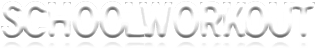Welcome to Schoolworkout/Maths

Key Stage 3 Mathematics:  For Students

 MATHSWORKOUT KS3 MATHS GCSE MATHS A LEVEL MATHS KS3 RESOURCES GCSE RESOURCES A LEVEL HOME

SUPPORT WORK

Some useful notes to support you through maths Key Stage 3 topics can be obtained by clicking on the relevant link below:

 Number topics Algebra topics Shape and space topics Data handling topics Introduction to percentages (level 6) Introduction to algebra (levels 5-6) Symmetry (pdf file) (level 5) Introduction to probability (levels 5-7) Fractions (levels 6-7) Inputs and outputs (expanding brackets) (levels 5-6) Area (levels 5-7) Introduction to averages (levels 5-7) Factors, multiples and prime factorisation  (level 7) Solving equations (unknowns on both sides) (level 6) Constructions (level 7) Percentage change (level 7)